## Introduction

In a modeling process, there are 3 core concepts that will always exist:

• Data.
• Features.
• Type of model and its corresponding parameters.

From data to the model, features are a measurable representation of the data, which would be the format for the data to be processed by the model thus method to create features from data is a must-to-do modeling process. Moreover, to improve model performance, apart from enhancing data quality and executing the model selection, having better features is also a possibility. On the other hand, the domain aspect related to the data information will affect the feature creation process by either being the requirement or providing the direction to execute it. Therefore, how to create features, do it well, and even relating domain knowledge to assist this process is a helpful skill to reach results easier.

This thus would lead us to the main purpose of this blog post: introduction to feature engineering. It is hoped that this blog could help in getting a sense of the operation and provide the start ground for anyone who would like to research feature engineering.

## What is feature engineering?

Feature engineering can be said as the process of transforming information from data into features that effectively enhance the model’s performance with the possibility of using domain knowledge as an assist.

As created features are not always useful and a large number of features could easily cause overfitting or curse of dimensionality situation, feature engineering would go along with feature selection to conclude only contributing features to be used for the model. Apart from that, regularization or kernel method can also help in limiting the growth of feature numbers effectively.

## Feature engineering process.

Since the modeling process is an iterative one, the feature engineering process is the same as well. The process can be summarized like this: after data gathering and preprocessing, data analysis and assumption can be used as the base to come up with the initial features set, and then after testing through the model result, a decision for further feature engineering or not can be made.

### The steps of a feature engineering process are:

• Looking at the data to have a direction for what features to start.
• Creating features.
• See the effectiveness of the feature provided for the result.
• If the result is still unsatisfied, evaluate and set a new direction for feature engineering.

# Mindset and consideration for feature engineering

### Mindset

Not only just for covering method to create features, but feature engineering principles has also evolved into constructing best practices and intuition in the feature creation process to avoid mistakes and boost the performance on a whole.

As data and use-case are situational, best feature engineering practices mostly can be achieved through tries out and analysis. There is no systematic way to do it, but underlying reasons do exist for practitioners to mainly do things in a certain way. The mindset for feature engineering is to actively operate experiments or study from past precedents to look for deeper principles and in the end shaping the intuition to execute the operation.

### Consideration

From “Feature Engineering and Selection: A Practical Approach for Predictive Models” (Kuhn & Johnson, 2019), some points worth noting:

• Overfitting is also a concern when a failed feature engineering introduces features that are relevant to the current dataset but don’t have any relationship with the outcomes when new additional data being included.
• To find the link between predictors and defined outcomes for prediction, supervised or unsupervised data analysis can be done.
• Considering the “No Free Lunch” Theorem, trying several of models’ types to see which one works best is the best course of action.
• Another concept that relating to this topic: Model vs modeling process, model bias and variance, experience-driven modeling & empirically driven modeling, and big data.

### They also showcase another example to present these points:

• The “Trial and error” process is needed to go through to find the best one.
• The interaction between models and features is complex and unpredictable. However, an effect from feature sets may be more significant than the effect of different models.
• Based on the same set of right features, the best performance can be ensured regardless of the model’s types.

## Feature engineering techniques’ introduction by following an example

Different data types will have their corresponding feature engineering techniques. This part of the blog post is to introduce the techniques by linking them with the data type so that when coming across the data type related techniques can be identified immediately.

# Introduction to Healthcare Data Science (Overview)

Healthcare analytics is the collection and analysis of data in the healthcare field to study determinants of disease in human populations, identify and mitigate risk by predicting outcomes. This post introduces  some common epidemiological study designs and an overview of the modern healthcare data analytics process.

# Types of Epidemiologic Studies

In general, epidemiologic studies can be classified into 3 types: Interventional study, Observational study, Meta-analysis study

## Interventional or Randomized control study

Clinical medicine relies on evidence base found in strong research to inform best practices and improve clinical care. The gold standard for study design to provide evidence is Randomized control (RCT). The main idea of this kind of research is to prove the root cause that makes a certain disease happen or the causal effect of a treatment. RCTs are performed in fairly homogeneous patient populations when participants are allocated by chance to two similar groups. The researchers then try different interventions or treatments for these two groups and compare the outcomes.

As an example, a study was conducted to assess whether improved lifestyle habits could reduce the hemoglobin A1c (HbA1c) levels of employees. In the experiment, the intervention consisted of a 3-month competition among employees to adopt  healthier lifestyle habits (Eat better, Move more, and Quit smoking) or keep their current lifestyle. After the intervention, employees with elevated HbA1c significantly reduced their HbA1c levels while employees without elevated HbA1c levels of employees without intervention were not changed.

In ideal conditions, there are no confounding variables in a randomized experiment, thus RCTs are often designed to investigate the causal relationship between exposure and outcome. However, RCTs have several limitations, RCTs are often costly, time-intensive, labor-intensive, slow, and can consist of homogeneous patients that are seldom generalizable to every patient population.

## Observational studies

Unlike RCTs, Observational studies have no active interventions which mean the researchers do not interfere with their participants. In contrast with interventional studies, observation studies are usually performed in heterogeneous patient populations. In these studies, researchers often define an outcome of interest (e.g a disease) and use data collected on patients such as demographic, labs, vital signs, and disease states to explore the relationship between exposures and outcome, determine which factors contributed to the outcome and attempt to draw inferences about the effects of different exposures on the outcome. Findings from observational studies can subsequently be developed and tested with the use of RCTs in targeted patient populations.

Observational studies tend to be less time- and cost-intensive. There are three main study designs in observational studies: prospective study design, retrospective study design, and cross-sectional study design.

### Follow-up study/ Prospective study/ Longitudinal (incidence) study

A prospective study is a study in which a group of disease-free individuals is identified as a baseline and are followed over some time until some of them develop the disease. The development of disease over time is then related to other variables measured at baseline, generally called exposure variables. The study population in a prospective study is often called a cohort.

### Retrospective study/ Case-Control study

A retrospective study is a study in which two groups of individuals are initially identified: (1) a group that has the disease under study (the cases) and (2) a group that does not have the disease under study (the controls). Cases are individuals who have a specific disease investigated in the research. Controls are those who did not have the disease of interest in the research. Usually, a retrospective history of health habits before getting the disease is obtained. An attempt is then made to relate their prior health habits to their current disease status. This type of study is also sometimes called a case-control study.

### Cross-sectional (Prevalence) study/ Prevalence study

A cross-sectional study is one in which a study population is ascertained at a single point in time. This type of study is sometimes called a prevalence study because the prevalence of disease at one point in time is compared between exposed and unexposed individuals. . Prevalence of a disease is obtained by dividing the number of people who currently have the disease by the number of people in the study population.

## Meta-data analysis

Often more than one investigation is performed to study a particular research question, by different research groups reporting significant differences for a particular finding and other research groups reporting no significant differences. Therefore, in meta-analysis researchers collect and synthesize findings from many existing studies and provides a clear picture of factors associated with the development of a certain disease. These results may be utilized for ranking and prioritizing risk factors in other researches.

# Modern Healthcare Data analytics approach

## Secondary Analysis and modern healthcare data analytics approach

In primary research infrastructure, designing a large-scale randomized controlled trial (RCTs) is expensive and sometimes unfeasible.  The alternative approach for expansive data is to utilize electronic health records (EHR). In contrast with the primary analysis, secondary analysis performs retrospective research using data collected for purposes other than research such as Electronic Health Record (EHR). Modern healthcare data analytic projects apply advanced data analysis methods, such as machine learning, and perform integrative analysis to leverage a wealth of deep clinical and administrative data with longitudinal history from EHR to get a more comprehensive understanding of the patient’s condition.

## Electronic Health Record (EHR)

EHRs are data generated during routine patient care. Electronic health records contain large amounts of longitudinal data and a wealth of detailed clinical information. Thus, the data, if properly analyzed and meaningfully interpreted, could vastly improve our conception and development of best practices. Common data in EHR are listed as the following:

• ### Demographics

Age, gender, occupation, marital status, ethnicity

• ### Physical measurement

SBP, DBP, Height, Weight, BMI, waist circumference

• ### Anthropometry

Stature, sitting height, elbow width, weight, subscapular, triceps skinfold measurement

• ### Laboratory

Creatinine, hemoglobin, white blood cell count (WBC), total cholesterol, cholesterol, triglyceride, gamma-glutamyl transferase (GGT)

• ### Symptoms

frequency in urination, skin rash, stomachache, cough

• ### Medical history and Family diseases

diabetes, traumas, dyslipidemia, hypertension, cancer, heart diseases, stroke, diabetes, arthritis, etc

• ### Lifestyle habit

Behavior risk factors from Questionnaires such as Physical activity, dietary habit, smoking, drinking alcohol, sleeping, diet, nutritional habits, cognitive function, work history, and digestive health, etc

• ### Treatment

Medications (prescriptions, dose, timing), procedures, etc.

# Using EHR to Conduct Outcome and Health Services Research

In the secondary analysis, the process of analyzing data often includes steps:

1. Problem Understanding and Formulating the Research Question: In this step, the process of transforming a clinical question into research is defined. There are 3 key components of the research question: the study sample (or patient cohort), the exposure of interest (e.g., information about patient demographic, lifestyle habit, medical history, regular health checkup test result), and the outcome of interest (e.g., a patient has diabetes or not after 5 years):
2. Data Preparation and Integration: Extracted raw data can be collected from different data sources, or be in separate datasets with different representation and formats. Data Preparation and Integration is the process of combining and reorganizing data derived from various data sources (such as databases, flat files, etc.) into a consistent dataset that contains all the information required for desired statistical analysis
3. Exploratory Data Analysis/ Data Understanding: Before statistics and machine learning models are employed, there is an important step of exploring data which is important for understanding the type of information that has been collected and what they mean. Data Exploration consists of investigating the distribution of variables, patterns, and nature inside the data and checking the quality of the underlying data. This preliminary examination will influence which methods will be most suitable for the data preprocessing step and choosing the appropriate predictive model
4. Data Preprocessing: Data preprocessing is one of the most important steps and critical in the success of machine learning techniques. Electronic health records (EHR) often were collected for clinical purposes. Therefore, these databases can have many data quality issues. Preprocessing aims at assessing and improving the quality of data to allow for reliable statistical analysis.
5. Feature Selection: Since the final dataset may have several hundreds of data fields, and not all of them are relevant to explain the target variable. In many machine learning algorithms, high-dimensionality can cause overfitting or reduce the accuracy of the model instead of improving it. Features selection algorithms are used to identify features that have an important predictive role. These techniques do not change the content of the initial features set, only select a subset of them. The purpose of feature selection is to help to create optimize and cost-benefit models for enhancing prediction performance.
6. Predictive Model: To develop prediction models with statistical models and machine learning algorithms could be employed. The purpose of machine learning is to design and develop prediction models, by allowing the computer to learn from data or experience to solve a certain problem. These models are useful for understanding the system under study, the models can be divided according to the type of outcome that they produce which includes the Classification model, Regression model, or Clustering model
7. Prediction and Model Evaluation: This process is to evaluate the performance of predictive models. The evaluation should include internal and external validation. Internal validation refers to the model performance evaluation in the same dataset in which the model was developed. External validation is the evaluation of a prediction model in other populations with different characteristics to assess the generalizability of the model.
Please also check our Healthcare Data Science example

# References:

 Fundamentals of Biostatistics – Bernard Rosner, Harvard University

 Secondary Analysis of Electronic Health Records – Springer Open

# Hiring Data Scientist / Engineer

We are looking for Data Scientist and Engineers.

# Vietnam AI / Data Science LabPlease also visit Vietnam AI Lab

# Introduction

With data that have time-related information, time features can be created to possibly add more information to the models.

Since how to consider time series for machine learning is a broad topic, this article only aims to introduced basic ways to create time features for those models.

## Type of data that is expected for this application

It is expected that transaction data type or any kind that similar to it will be the common one for this application. Other kinds of data that have timestamps information for each data point should also apply to some extent of this approach well.

## Considering before attempt: a need of analyzing the problem and scope

For data with the time element, it can be presented as a time series, which is how a set of data points described by an entity are follow ordered indexes of time. One aspect considered for time series is that observations are expected to depend on the previous one in time sequence, with the latter is correlated to the one before. In those cases, using time series models for forecasting is a straightforward approach to use this data. Another way to approach it is to use feature engineering to transform data to have features that can be used for supervised machine learning models, which is the focus of this article.

Using the time series model or adapting the machine learning model is dependent. In some cases, domain knowledge or business requirement will influence this decision.  It is better to analyze the problem first to see the need of using either one or both types of models.

Regardless of the domain knowledge  or business requirement aspects, the approach decision should have always considering the efficiency the approach will bring in terms of accuracy and computation cost.

# Basic methods

## A first preprocessing step to have the first set of time features: extracting time information from timestamp

The most straightforward thing to do is to extract basic time units, which for instance are hours, date, month, years into separates features. Another kind of information that can also be extracted is the characteristic of the time, which could be whether the time is at a part of days (morning, afternoon), is weekend or not or is it a holiday, etc.

In some business requirements or domains’ aspects, those initial features at this level are already needed to see if the value of observation is followed those factors or not. For example, the data is the record of timestamps of customers visiting a shop and their purchases. There is a need to know at which hours, date, month… a customer would come and purchase so that follow-up actions can be made to increase sales.

## Aggregate techniques

Regarding feature engineering for time data, the well-known technique that is commonly used is aggregate features by taking statistics (variance, max, min, etc.) of the set of values grouped by the desired time unit: hours, days, months…

Apart from that, a time window could be defined and compute aggregate by rolling or expanding the time window.

• Rolling: have a fixed time window size and to predict a value for the data point at a time, features will be computed from aggregating backward number of time steps according to the time window
• Expanding: from the data point, the window will be the whole record of past time steps.

There are also two aspects of aggregating:

• Aggregating to create new features for the current data points. For the first case, the model is considered to include the time series characteristic, meaning a moment will likely be related to other moments from the recent past.
• Aggregating to create a new set of data points with a corresponding new set of features from the current ones. For the second one, the new number of data points considering for the model is changed and each new data point is the summary of information from a subset of initial data points. As a result, objects for the models may be shifted like being mentioned in considering the before part. If the data only about the information record of one entity, or in other words only contains one time series of an entity, through this technique, the new computed data points can be the summary of other features’ value in the chosen time unit. On the other hand, if there are more entities observed in the data set, each new data point is then the summary information of each observed entity.

How to decide on the focus objects for the problem and the approach is situational but for a fresh problem and fresh data with no specific requirement or prior domain knowledge, it is better to consider all of them for the model and execute feature selection to see if the created time features are of any value.

# Dealing with hours of a day – Circular data

For some needs, a specific time of the day is required to be focus. A use case for detecting fraud transactions is a good example of this. To find something like the most frequent time that a kind of behavior is performed, for instance, using the arithmetic mean may be misleading and is not a good representation. An important point that needs to be considered is that hours of the day is a circular data and it should be represented on a circular axis with its’ value between 0 to 2π. To have a better representation of the mean, using von Mises distribution to have periodic mean is a suitable approach for this situation (Mishtert, 2019).

# Validation for the model

Before the model building, a validation set is needed to be selected from the data first. In the usual cases, to avoid overfitting data will be randomly shuffled and then will be divided into a training set and validation set. However, for this kind of situation, it shouldn’t be done so to avoid the mistake of having past data in the validation and the future data in the training, in other words using future data to predict the past.

## k-Nearest Neighbors algorithms

In this blog post, I am going to introduce one of the most intuitive algorithms in the field of Supervised Learning, the k-Nearest Neighbors algorithm (kNN).

# The original k-Nearest Neighbors algorithm

The kNN algorithm is very intuitive. Indeed, with the assumption that items close together in the dataset are typically similar, kNN infers the output of a new sample by first constructing the distance score with every sample in the training dataset. From there, it creates a ‘neighbor zone’ by selecting samples that are ‘near’ the candidate one, and does the supervised tasks based on samples lie inside that zone. The task could be either classification or regression.

Let’s start with the basic kNN algorithm. Letbe our training dataset withsamples belong toclasses, whereis the class of one sample, anddenotes the corresponding feature vector that describes the characteristics of that sample. Furthermore, it is necessary to define the suitable distance metric, since it drives the algorithm to select neighbors and make predictions later on. The distance metric, is a mappingover a vector space, where the following conditions are satisfied:

••••In the following steps to describe the k-Nearest Neighbors algorithm, the Euclidean distance will be used as the distance metric.

For any new instance:

• Findwhereis the set ofsamples that are closest to• The way to define the nearest neighbors is based on distance metric(Note that we are using Euclidean distance).• The classifieris defined as:whereis the unit function. Note that for the regression problem, the functionwill just an average of all response valuesfrom neighbor samples.

# Weighted k-Nearest Neighbors

In the kNN algorithm, we weigh all neighbors equally. It may affect the inference steps, especially when the neighbor zone becomes bigger and bigger. To strengthen the effect of ‘close’ neighbors than others, the weighted scheme of k-Nearest Neighbors is applied.

Weighted k-Nearest Neighbors is based on the idea that, within, such observations that are closer to, should get a higher weight than the further neighbors. Now it is necessary to note some properties of any weighting schemeson any distance metric:

•••decreases monotonously forFor any new instance:

• We findwhereis the set ofsamples that are closest to• Theth neighbor is used for standardization of thesmallest distance:• We transform the standardized distancewith any kernel functioninto weights.
• The classifieris defined as:# The pros and cons of kNN, and further topics

The kNN and weighted kNN do not rely on any specific assumption on the distribution of the data, so it is quite easy to apply it to many problems as the baseline model. Furthermore, kNN (and its family) is very intuitive for understanding and implementing, which again makes it a worthy try-it-first approach for many supervised problems.

Despite those facts, kNN still has challenges in some aspects: It is computationally expensive – especially when the dataset size becomes huge. Another challenge is choosing the ‘correct’ distance metric that best follows the assumption for using this algorithm: items close together in the data set should be typically similar. Lastly, the curse of dimensionality heavily affects the distance metric. Beyer et al. proves that, under some preconditions, in high dimension space, all points converge to the same distance from the query point. In this case, the concept of ‘nearest neighbors’ is no longer meaningful.

# I. A Brief Overview

Consider an example of a courtroom trial:

A car company C is accused of not manufacturing environment-friendly vehicles. The average CO2 emission per car from different manufacturers based on a survey from the previous year is 120.4 grams per kilometer. But for a random batch of 100 cars produced at C’s factory, the average CO2 emission is 121.2 grams per kilometer with a standard deviation of 1.8.

At the trial, Company C is not considered to be guilty as long as their wrongdoing is not proven. A public prosecutor tries to prove that C is guilty and can only succeed when enough evidence is presented.

The example above illustrates the concepts of hypothesis testing; specifically, there are two conflicting hypotheses:

i) C is not guilty; or

ii) C is guilty

The first is called the null hypothesis (denoted by H0), and the second the alternative hypothesis (denoted by HA). At the start of the trial, the null hypothesis is temporarily accepted, until proven otherwise. The goal of hypothesis testing is to perform some sort of transformed comparison between the two numbers 121.2 and 120.4 to either reject H0 and accept HA or vice versa. This one-sample mean testing because we are comparing the average value obtained from one sample (121.2) with the average value assumed to represent the whole population (120.4)

# II. Required Steps for Hypothesis Testing

The six steps below must be followed to conduct a hypothesis test. The details will be elaborated on with our example afterward.

1) Set up null and alternative hypotheses and check conditions.

2) Determine the significance level, alpha.

3) Calculate the test statistic.

4) Calculate the probability value (a.k.a the p-value), or find the rejection region. For the following example, we will use the p-value.

5) Decide on the null hypothesis.

6) State the overall conclusion.

# III. A step-by-step example

## 1) Set up hypotheses:

We already mentioned in the beginning the two hypotheses. But now we will formalize them:

### Null hypothesis:

Company C’s CO2 mean (denoted by μ ) is equal to the population mean (denoted by μ0):           μ = μ0

### Alternative hypothesis:

Company C’s CO2 mean is greater than the population mean: μ > μ0

The hypothesis testing for the one-sample means we are conducting requires the data to come from an approximately normal distribution or a large enough sample size, which can be quite subjective. To keep things simple, we decide that the data gathered from company C is big enough with the sample size being 100 cars.

## 2) Determine the significance level, alpha, or confidence level

The significance level and its complementary, the confidence level, provide a level of probability cutoff for our test to make decisions about the hypotheses. A common value for alpha is 5%, which is the same as a confidence level of 95%.

## 3) Calculate the test statistic

For the one-sample mean test, we calculate the t* test statistic using the formula: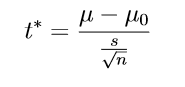where s is the standard deviation from the sample we are testing, 1.8, and n is the size of the sample, 100.

## Binomial Theorem

Can you expand on? I guess you would find that is quite easy to do. You can easily find that.

How about the expansion of. It is no longer easy.

It is no longer easy, isn’t it. However, if we use Binomial Theorem, this expansion becomes an easy problem.

Binomial Theorem is a very intriguing topic in mathematics and it has a wide range of applications.

# Theorem

Letbe real numbers (or complex, or polynomial). For any positive integer, we have:where,Proof:

We will use prove by induction. The base caseis obvious. Now suppose that the theorem is true for the case, that is assume that:we will need to  show that, this is true forLet us consider the left-hand side of the equation above## We can now apply Pascal’s identity:The equation above can be simplified to:as we desired.

### Example 1:  Power rule in Calculus

In calculus, we always use the power rule thatWe can prove this rule using the Binomial Theorem.

Proof:

Recall that derivative for any continuous function f(x) is defined as:Letbe a positive integer and letThe derivative of f(x) is:### Example 2:  Binomial Distribution

Let X be the number of Head a sequence of n independent coin tossing. X is usually model by binomial distribution in the probability model. Letbe the probability that a head shows up in a toss, and let. The probability that there ishead in the sequence oftoss is:We know that sum of all the probability must equal to 1. In order to show this, we can use Binomial Theorem. We have:Please also check another article Gaussian Samples and N-gram language models ,Bayesian model, Monte Carlo for statistics knowledge.

# Hiring Data Scientist / Engineer

We are looking for Data Scientist and Engineer.

# Vietnam AI / Data Science LabPlease also visit Vietnam AI Lab

## Monte Carlo Simulation

On a nice day 2 years ago, when I was in the financial field. My boss sent our team an email. In this email, he would like to us propose some machine learning techniques to predict stock price.

So, after accepting the assignment from my manager, our team begin to research and apply some approaches for prediction. When we talk about Machine Learning, we often think of supervised and unsupervised learning. But one of the algorithms we applied is one that we forgot however equally highly effective algorithm: Monte Carlo Simulation.

# What is Monte Carlo simulation?

The Monte Carlo method is a technique that uses random numbers and probability to solve complex problems. The Monte Carlo simulation, or probability simulation, is a technique used to understand the impact of risk and uncertainty in financial sectors, project management, costs, and other forecasting machine learning models.

Now let’s jump into python implementation to see how it applies,

# Python Implementation

In this task, we used data of DXG stock dataset from 2017/01/01 to 2018/08/24 and we would like to know what is stock price after 10 days, 1 month, and 3 months, respectively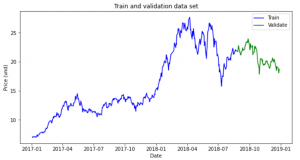We will simulate the return of stock and next price will be calculated by

P(t) = P(0) * (1+return_simulate(t))

Calculate mean and standard deviation of stock returns

miu = np.mean(stock_returns, axis=0)
dev = np.std(stock_returns)

Simulation process

simulation_df = pd.DataFrame()
last_price = init_price
for x in range(mc_rep):
count = 0
daily_vol = dev
price_series = []
price = last_price * (1 + np.random.normal(miu, daily_vol))
price_series.append(price)
for y in range(train_days):
if count == train_days-1:
break
price = price_series[count] * (1 + np.random.normal(miu, daily_vol))
price_series.append(price)
count += 1
simulation_df[x] = price_series

Visualization Monte Carlo Simulation

fig = plt.figure()
fig.suptitle('Monte Carlo Simulation')
plt.plot(simulation_df)
plt.axhline(y = last_price, color = 'r', linestyle = '-')
plt.xlabel('Day')
plt.ylabel('Price')
plt.show()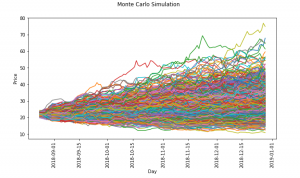Now, let’s check with actual stock price after 10 days, 1 month and 3 months

plt.hist(simulation_df.iloc[9,:],bins=15,label ='histogram')
plt.axvline(x = test_simulate.iloc, color = 'r', linestyle = '-',label ='Price at 10th')
plt.legend()
plt.title('Histogram simulation and last price of 10th day')
plt.show()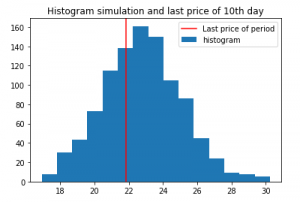We can see the most frequent occurrence price is pretty close to the actual price after 10th

If the forecast period is longer, the results are not good gradually

Simulation for next 1 month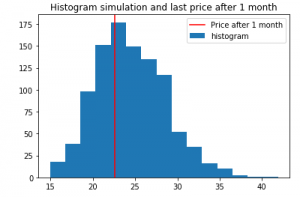After 3 months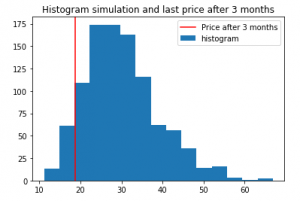# Conclusion

Monte Carlo simulation is used a lot in finance, although it has some weaknesses, hopefully through this article, you will have a new look at the simulation application for forecasting.

# Reference

 Pratik Shukla, Roberto Iriondo, “Monte Carlo Simulation An In-depth Tutorial with Python”, medium, https://medium.com/towards-artificial-intelligence/monte-carlo-simulation-an-in-depth-tutorial-with-python-bcf6eb7856c8

Please also check Gaussian Samples and N-gram language models,
Bayesian Statistics for more statistics knowledge.

# Hiring Data Scientist / Engineer

We are looking for Data Scientist and Engineer.

# Vietnam AI / Data Science LabPlease also visit Vietnam AI Lab

## Bayesian estimator of the Bernoulli parameter

In this post, I will explain how to calculate a Bayesian estimator. The taken example is very simple: estimate the parameter θ of a Bernoulli distribution.

A random variable X which has the Bernoulli distribution is defined as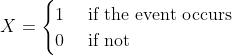with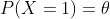In this case, we can write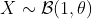.

In reality, the simplest way to estimate θ is to sample X, count how many times the event occurs, then estimate the probability of occurring events. This is exactly what the frequentists do.

In this post, I will show how do the Bayesian statisticians estimate θ. Although this doesn’t have a meaningful application, it helps to understand how do Bayesian statistics work. Let’s start.

# The posterior distribution of θ

Denote Y as the observation of the event. Given the parameter θ, if we sample the event n time, then the probability that the event occurs k time is (this is called the probability density function of Bernoulli )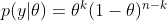In Bayesian statistics, we would like to calculate

By using the Bayesian formula, we haveWith the prior distribution of theta as a Uniform distribution, p(θ) = 1, and it is easy to prove thatwhere Γ is the Gamma distribution. Hence, the posterior distribution isFortunately, this is the density function of the Beta distribution:We use the following properties for evaluating the posterior mean and variance of theta.

If, then# Simulation

In summary, the Bayesian estimator of theta is the Beta distribution with the  mean and variance as above. Here are the Python codes for simulating data and estimating theta

def bayes_estimator_bernoulli(data, a_prior=1, b_prior=1, alpha=0.05):
'''Input:
data is a numpy array with binary value, which has the distribution B(1,theta)    a_prior, b_prior: parameters of prior distribution Beta(a_prior, b_prior)    alpha: significant level of the posterior confidence interval for parameter    Model:
for estimating the parameter theta of a Bernoulli distribution    the prior distribution for theta is Beta(1,1)=Uniform[0,1]    Output:
a,b: two parameters of the posterior distribution Beta(a,b)
pos_mean: posterior estimation for the mean of theta
pos_var: posterior estimation for the var of theta'''
n = len(data)
k = sum(data)
a = k+1
b = n-k+1
pos_mean = 1.*a/(a+b)
pos_var = 1.*(a*b)/((a+b+1)*(a+b)**2)
## Posterior Confidence Interval
theta_inf, theta_sup = beta.interval(1-alpha,a,b)
print('Prior distribution: Beta(%3d, %3d)' %(a_prior,b_prior))
print('Number of trials: %d, number of successes: %d' %(n,k))
print('Posterior distribution: Beta(%3d,%3d)' %(a,b))
print('Posterior mean: %5.4f' %pos_mean)
print('Posterior variance: %5.8f' %pos_var)
print('Posterior std: %5.8f' %(np.sqrt(pos_var)))
print('Posterior Confidence Interval (%2.2f): [%5.4f, %5.4f]' %(1-alpha, theta_inf, theta_sup))
return a, b, pos_mean, pos_var

# Example
n = 129 # sample size
data = np.random.binomial(size=n, n=1, p=0.6)
a, b, pos_mean, pos_var = bayes_estimator_bernoulli(data)

And the result is

Prior distribution: Beta(  1,   1)
Number of trials: 129, number of successes: 76
Posterior distribution: Beta( 77, 54)
Posterior mean: 0.5878
Posterior variance: 0.00183556
Posterior std: 0.04284341
Posterior Confidence Interval (0.95): [0.5027, 0.6703]

In the simulation, we simulated 129 data from the Bernoulli distribution with θ=0.6. And the Bayesian estimation of θ is the posterior mean which is 0.5878.
This is a very simple example of Bayesian estimation. In reality, it is usually tricky to determine a closed-form solution of the posterior distribution from the given prior distribution. In that case, the Monte Carlo technique is one of the solutions to approximate the posterior distribution.
Please also check Gaussian Samples and N-gram language models for more statistics knowledge.

# Hiring Data Scientist / Engineer

We are looking for Data Scientist and Engineer.

# Vietnam AI / Data Science LabPlease also visit Vietnam AI Lab

# Background

In part 1 of my project, I built a unigram language model: it estimates the probability of each word in a text simply based on the fraction of times the word appears in that text.

The text used to train the unigram model is the book “A Game of Thrones” by George R. R. Martin (called train). The texts on which the model is evaluated are “A Clash of Kings” by the same author (called dev1), and “Gone with the Wind” — a book from a completely different author, genre, and time (called dev2).

In this part of the project, I will build higher n-gram models, from bigram (n=2) to 5-gram (n=5). These models are different from the unigram model in part 1, as the context of earlier words is taken into account when estimating the probability of a word.

# Higher n-gram language models

## Training the model

For a given n-gram model:

The example below shows how to calculate the probability of a word in a trigram model: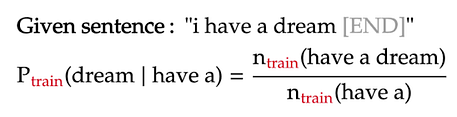For simplicity, all words are lower-cased in the language model, and punctuations are ignored. The presence of the [END] tokens is explained in part 1.

# Dealing with words near the start of a sentence

In higher n-gram language models, the word near the start of each sentence will not have a long enough context to apply the formula above. To make the formula consistent for those cases, we will pad these n-grams with sentence-starting symbols [S]. Below are two such examples under the trigram model:

From the above formulas, we see that the n-grams containing the starting symbols are just like any other n-gram. The only difference is that we count them only when they are at the start of a sentence. Lastly, the count of n-grams containing only [S] symbols is naturally the number of sentences in our training text:

# Dealing with unknown n-grams

Similar to the unigram model, the higher n-gram models will encounter n-grams in the evaluation text that never appeared in the training text. This can be solved by adding pseudo-counts to the n-grams in the numerator and/or denominator of the probability formula a.k.a. Laplace smoothing. However, as outlined in part 1 of the project, Laplace smoothing is nothing but interpolating the n-gram model with a uniform model, the latter model assigns all n-grams the same probability: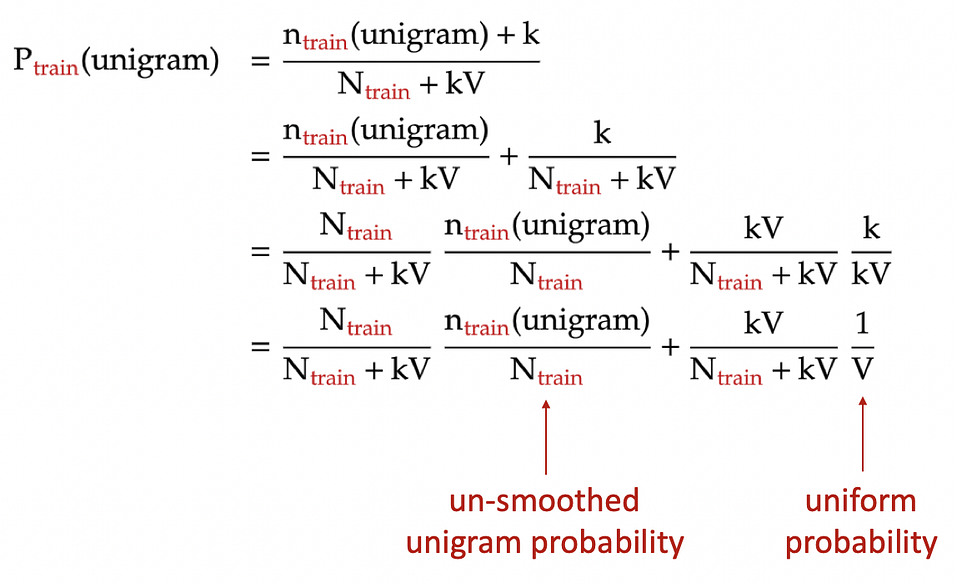Laplace smoothing for unigram model: each unigram is added a pseudo-count of k. N: total number of words in the training text. V: number of unique unigrams in the training text.

Hence, for simplicity, for an n-gram that appears in the evaluation text but not the training text, we just assign zero probability to that n-gram. Later, we will smooth it with the uniform probability.

# Background

Language modeling — that is, predicting the probability of a word in a sentence — is a fundamental task in natural language processing. It is used in many NLP applications such as autocompletespelling correction, or text generation.

Currently, language models based on neural networks, especially transformers, are the state of the art: they predict very accurately a word in a sentence based on surrounding words. However, in this project, I will revisit the most classic language model: the n-gram models.

# Data

In this project, my training data set — appropriately called train — is “A Game of Thrones”, the first book in the George R. R. Martin fantasy series that inspired the popular TV show of the same name.

Then, I will use two evaluating texts for our language model:

# Unigram language model

## What is a unigram?

In natural language processing, an n-gram is a sequence of n words. For example, “statistics” is a unigram (n = 1), “machine learning” is a bigram (n = 2), “natural language processing” is a trigram (n = 3), and so on. For longer n-grams, people just use their lengths to identify them, such as 4-gram, 5-gram, and so on. In this part of the project, we will focus only on language models based on unigrams i.e. single words.

## Training the model

A language model estimates the probability of a word in a sentence, typically based on the words that have come before it. For example, for the sentence “I have a dream”, our goal is to estimate the probability of each word in the sentence based on the previous words in the same sentence: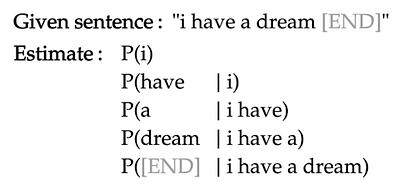For simplicity, all words are lower-cased in the language model, and punctuations are ignored. The [END] token marks the end of the sentence and will be explained shortly.

The unigram language model makes the following assumptions: